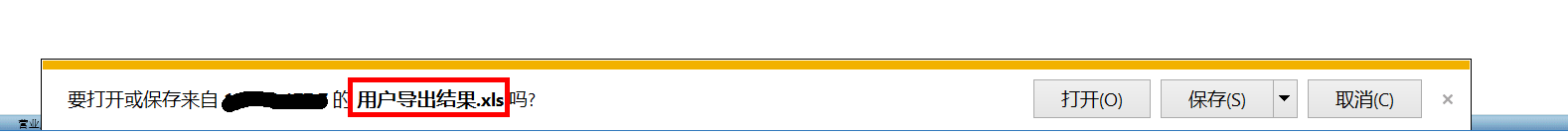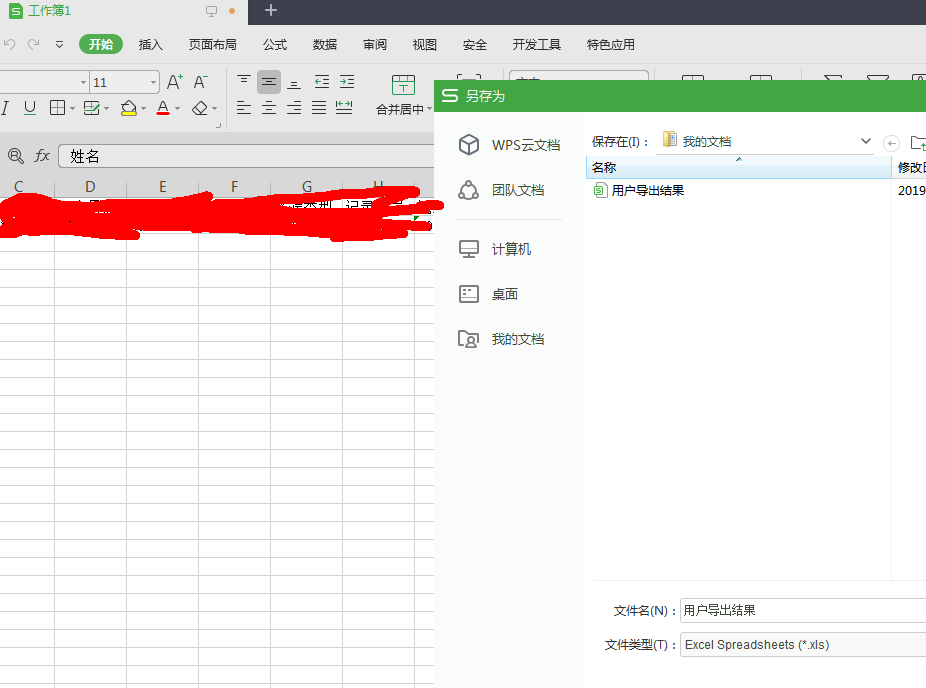/***导出勾选列内容生成excel***/
function exportToExcel(tableid,chkname) //读取表格中每个单元到EXCEL中
{

`````` var curTbl = document.getElementById(tableid);
if(chkname==undefined) chkname="";
var curChk = \$("[name='"+chkname+"']");
var oXL;

try{
oXL = new ActiveXObject("Excel.Application"); //创建AX对象excel
}catch(e){
dalert("无法启动Excel!\n\n如果您确信您的电脑中已经安装了Excel，"+"那么请调整IE的安全级别。\n\n具体操作：\n\n"+"工具 → Internet选项 → 安全 → 自定义级别 → 对没有标记为安全的ActiveX进行初始化和脚本运行 → 启用");
return false;
}

var oSheet = oWB.ActiveSheet;//激活当前sheet
var hang = curTbl.rows.length;//取得表格行数
var lie =curTbl.rows(0).cells.length;//取得数据源列数
for(var i=0;i<hang;i++){//i为表单对应的行，j为表单对应的列
for(var j=0,k=0;j<lie-1;j++,k++){//k为生成EXCEL对应的列

if(curChk[j].checked){//当勾选上时记录相应的数据
oSheet.Cells(i+1,k+1).NumberFormatLocal = "@";//设置单元格数字格式为文本
oSheet.Cells(i+1,k+1).value=curTbl.rows(i).cells(j).innerText;
}else{
k--;//当未勾选时，EXCEL列回退
}
}
}
oXL.Visible = true;//设置excel可见属性
try{
var fname = oXL.Application.GetSaveAsFilename("用户导出结果.xls", "Excel Spreadsheets (*.xls), *.xls");
}catch(e){
print("Nested catch caught " + e);
}finally{
location.href='用户导出结果.xls';
oWB.SaveAs(fname);

oWB.Close(savechanges=false);
//xls.visible = false;
oXL.Quit();
oXL=null;
//结束excel进程，退出完成
//window.setInterval("Cleanup();",1);
idTmr = window.setInterval("Cleanup();",1);
}
``````

}

IE浏览器
1.我想要的是这种展示：（这是用jsp中java代码写的输出流）2.目前js导出的，就直接把excel打开了，又弹出了另存为。是这样的：（现在只用js，不能像之前那样）（查了很久，貌似用a标签路径下载可以，但我是ajax查询返回的数据，在调用
exportToExcel方法，如何实现）